# mixconjugateblm

Bayesian linear regression model with conjugate priors for stochastic search variable selection (SSVS)

## Description

The Bayesian linear regression model object mixconjugateblm specifies the joint prior distribution of the regression coefficients and the disturbance variance (β, σ2) for implementing SSVS (see  and ) assuming β and σ2 are dependent random variables.

In general, when you create a Bayesian linear regression model object, it specifies the joint prior distribution and characteristics of the linear regression model only. That is, the model object is a template intended for further use. Specifically, to incorporate data into the model for posterior distribution analysis and feature selection, pass the model object and data to the appropriate object function.

## Creation

### Syntax

PriorMdl = mixconjugateblm(NumPredictors)
PriorMdl = mixconjugateblm(NumPredictors,Name,Value)

### Description

example

 PriorMdl = mixconjugateblm(NumPredictors) creates a Bayesian linear regression model object (PriorMdl) composed of NumPredictors predictors and an intercept, and sets the NumPredictors property. The joint prior distribution of (β, σ2) is appropriate for implementing SSVS for predictor selection . PriorMdl is a template that defines the prior distributions and the dimensionality of β.

example

 PriorMdl = mixconjugateblm(NumPredictors,Name,Value) sets properties (except NumPredictors) using name-value pair arguments. Enclose each property name in quotes. For example, mixconjugateblm(3,'Probability',abs(rand(4,1))) specifies random prior regime probabilities for all four coefficients in the model.

## Properties

expand all

You can set writable property values when you create the model object by using name-value pair argument syntax, or after you create the model object by using dot notation. For example, to exclude an intercept from the model, enter

PriorMdl.Intercept = false;

Number of predictor variables in the Bayesian multiple linear regression model, specified as a nonnegative integer.

NumPredictors must be the same as the number of columns in your predictor data, which you specify during model estimation or simulation.

When specifying NumPredictors, exclude any intercept term for the value.

After creating a model, if you change the of value NumPredictors using dot notation, then these parameters revert to the default values:

• Variables names (VarNames)

• Prior mean of β (Mu)

• Prior variances of β for each regime (V)

• Prior correlation matrix of β (Correlation)

• Prior regime probabilities (Probability)

Data Types: double

Flag for including a regression model intercept, specified as a value in this table.

ValueDescription
falseExclude an intercept from the regression model. Therefore, β is a p-dimensional vector, where p is the value of NumPredictors.
trueInclude an intercept in the regression model. Therefore, β is a (p + 1)-dimensional vector. This specification causes a T-by-1 vector of ones to be prepended to the predictor data during estimation and simulation.

If you include a column of ones in the predictor data for an intercept term, then set Intercept to false.

Example: 'Intercept',false

Data Types: logical

Predictor variable names for displays, specified as a string vector or cell vector of character vectors. VarNames must contain NumPredictors elements. VarNames(j) is the name of the variable in column j of the predictor data set, which you specify during estimation, simulation, or forecasting.

The default is {'Beta(1)','Beta(2),...,Beta(p)}, where p is the value of NumPredictors.

Example: 'VarNames',["UnemploymentRate"; "CPI"]

Data Types: string | cell | char

Component-wise mean hyperparameter of the Gaussian mixture prior on β, specified as an (Intercept + NumPredictors)-by-2 numeric matrix. The first column contains the prior means for component 1 (the variable-inclusion regime, that is, γ = 1). The second column contains the prior means for component 2 (the variable-exclusion regime, that is, γ = 0).

• If Intercept is false, then Mu has NumPredictors rows. mixconjugateblm sets the prior mean of the NumPredictors coefficients corresponding to the columns in the predictor data set, which you specify during estimation, simulation, or forecasting.

• Otherwise, Mu has NumPredictors + 1 elements. The first element corresponds to the prior means of the intercept, and all other elements correspond to the predictor variables.

Tip

To perform SSVS, use the default value of Mu.

Example: In a 3-coefficient model, 'Mu',[0.5 0; 0.5 0; 0.5 0] sets the component 1 prior mean of all coefficients to 0.5 and sets the component 2 prior mean of all coefficients to 0.

Data Types: double

Component-wise variance factor hyperparameter of the Gaussian mixture prior on β, an (Intercept + NumPredictors)-by-2 positive numeric matrix. The first column contains the prior variance factors for component 1 (the variable-inclusion regime, that is, γ = 1). The second column contains the prior variance factors for component 2 (the variable-exclusion regime, that is, γ = 0). Regardless of regime or coefficient, the prior variance of a coefficient is the variance factor times σ2.

• If Intercept is false, then V has NumPredictors rows. mixconjugateblm sets the prior variance factor of the NumPredictors coefficients corresponding to the columns in the predictor data set, which you specify during estimation, simulation, or forecasting.

• Otherwise, V has NumPredictors + 1 elements. The first element corresponds to the prior variance factor of the intercept, and all other elements correspond to the predictor variables.

Tip

• To perform SSVS, specify a larger variance factor for regime 1 than for regime 2 (for all j, specify V(j,1) > V(j,2)).

• For more details on what value to specify for V, see .

Example: In a 3-coefficient model, 'V',[100 1; 100 1; 100 1] sets the component 1 prior variance factor of all coefficients to 100 and sets the component 2 prior variance factor of all coefficients to 1.

Data Types: double

Prior probability distribution for the variable inclusion and exclusion regimes, specified as an (Intercept + NumPredictors)-by-1 numeric vector of values in [0,1], or a function handle in the form @fcnName, where fcnName is the function name. Probability represents the prior probability distribution of γ = {γ1,…,γK}, where:

• K = Intercept + NumPredictors, which is the number of coefficients in the regression model.

• γk ∈ {0,1} for k = 1,…,K. Therefore, the sample space has a cardinality of 2K.

• γk = 1 indicates variable VarNames(k) is included in the model, and γk = 0 indicates that the variable is excluded from the model.

If Probability is a numeric vector:

• Rows correspond to the variable names in VarNames. For models containing an intercept, the prior probability for intercept inclusion is Probability(1).

• For k = 1,…,K, the prior probability for excluding variable k is 1 – Probability(k).

• Prior probabilities of the variable-inclusion regime, among all variables and the intercept, are independent.

If Probability is a function handle, then it represents a custom prior distribution of the variable-inclusion regime probabilities. The corresponding function must have this declaration statement (the argument and function names can vary):

logprob = regimeprior(varinc)

• logprob is a numeric scalar representing the log of the prior distribution. You can write the prior distribution up to a proportionality constant.

• varinc is a K-by-1 logical vector. Elements correspond to the variable names in VarNames and indicate the regime in which the corresponding variable exists. varinc(k) = true indicates VarName(k) is included in the model, and varinc(k) = false indicates it is excluded from the model.

You can include more input arguments, but they must be known when you call mixconjugateblm.

For details on what value to specify for Probability, see .

Example: In a 3-coefficient model, 'Probability',rand(3,1) assigns random prior variable-inclusion probabilities to each coefficient.

Data Types: double | function_handle

Prior correlation matrix of β for both components in the mixture model, specified as an (Intercept + NumPredictors)-by-(Intercept + NumPredictors) numeric, positive definite matrix. Consequently, the prior covariance matrix for component j in the mixture model is sigma2*diag(sqrt(V(:,j)))*Correlation*diag(sqrt(V(:,j))), where sigma2 is σ2 and V is the matrix of coefficient variance factors.

Rows and columns correspond to the variable names in VarNames.

By default, regression coefficients are uncorrelated, conditional on the regime.

Note

You can supply any appropriately sized numeric matrix. However, if your specification is not positive definite, mixconjugateblm issues a warning and replaces your specification with CorrelationPD, where:

CorrelationPD = 0.5*(Correlation + Correlation.');

For details on what value to specify for Correlation, see .

Data Types: double

Shape hyperparameter of the inverse gamma prior on σ2, specified as a numeric scalar.

A must be at least –(Intercept + NumPredictors)/2.

With B held fixed, the inverse gamma distribution becomes taller and more concentrated as A increases. This characteristic weighs the prior model of σ2 more heavily than the likelihood during posterior estimation.

For the functional form of the inverse gamma distribution, see Analytically Tractable Posteriors.

Example: 'A',0.1

Data Types: double

Scale parameter of inverse gamma prior on σ2, specified as a positive scalar or Inf.

With A held fixed, the inverse gamma distribution becomes taller and more concentrated as B increases. This characteristic weighs the prior model of σ2 more heavily than the likelihood during posterior estimation.

Example: 'B',5

Data Types: double

## Object Functions

 estimate Perform predictor variable selection for Bayesian linear regression models simulate Simulate regression coefficients and disturbance variance of Bayesian linear regression model forecast Forecast responses of Bayesian linear regression model plot Visualize prior and posterior densities of Bayesian linear regression model parameters summarize Distribution summary statistics of Bayesian linear regression model for predictor variable selection

## Examples

collapse all

Consider the linear regression model that predicts the US real gross national product (GNPR) using a linear combination of industrial production index (IPI), total employment (E), and real wages (WR).

${\text{GNPR}}_{t}={\beta }_{0}+{\beta }_{1}{\text{IPI}}_{t}+{\beta }_{2}{\text{E}}_{t}+{\beta }_{3}{\text{WR}}_{t}+{\epsilon }_{t}.$

For all $t$, ${\epsilon }_{t}$ is a series of independent Gaussian disturbances with a mean of 0 and variance ${\sigma }^{2}$.

Assume these prior distributions for $\mathit{k}$ = 0,...,3:

• ${\beta }_{k}|{\sigma }^{2},{\gamma }_{k}={\gamma }_{k}\sigma \sqrt{{V}_{k1}}{Z}_{1}+\left(1-{\gamma }_{k}\right)\sigma \sqrt{{V}_{k2}}{Z}_{2}$, where ${\mathit{Z}}_{1}$ and ${\mathit{Z}}_{2}$ are independent, standard normal random variables. Therefore, the coefficients have a Gaussian mixture distribution. Assume all coefficients are conditionally independent, a priori, but they are dependent on the disturbance variance.

• ${\sigma }^{2}\sim IG\left(A,B\right)$. $A$ and $B$ are the shape and scale, respectively, of an inverse gamma distribution.

• ${\gamma }_{\mathit{k}}\in \left\{0,1\right\}$and it represents the random variable-inclusion regime variable with a discrete uniform distribution.

Create a prior model for SSVS. Specify the number of predictors p.

p = 3; PriorMdl = mixconjugateblm(p);

PriorMdl is a mixconjugateblm Bayesian linear regression model object representing the prior distribution of the regression coefficients and disturbance variance. mixconjugateblm displays a summary of the prior distributions at the command line.

Alternatively, you can create a prior model for SSVS by passing the number of predictors to bayeslm and setting the ModelType name-value pair argument to 'mixconjugate'.

MdlBayesLM = bayeslm(p,'ModelType','mixconjugate')
MdlBayesLM = mixconjugateblm with properties: NumPredictors: 3 Intercept: 1 VarNames: {4x1 cell} Mu: [4x2 double] V: [4x2 double] Probability: [4x1 double] Correlation: [4x4 double] A: 3 B: 1 | Mean Std CI95 Positive Distribution ------------------------------------------------------------------------------ Intercept | 0 1.5890 [-3.547, 3.547] 0.500 Mixture distribution Beta(1) | 0 1.5890 [-3.547, 3.547] 0.500 Mixture distribution Beta(2) | 0 1.5890 [-3.547, 3.547] 0.500 Mixture distribution Beta(3) | 0 1.5890 [-3.547, 3.547] 0.500 Mixture distribution Sigma2 | 0.5000 0.5000 [ 0.138, 1.616] 1.000 IG(3.00, 1) 

Mdl and MdlBayesLM are equivalent model objects.

You can set writable property values of created models using dot notation. Set the regression coefficient names to the corresponding variable names.

PriorMdl.VarNames = ["IPI" "E" "WR"]
PriorMdl = mixconjugateblm with properties: NumPredictors: 3 Intercept: 1 VarNames: {4x1 cell} Mu: [4x2 double] V: [4x2 double] Probability: [4x1 double] Correlation: [4x4 double] A: 3 B: 1 | Mean Std CI95 Positive Distribution ------------------------------------------------------------------------------ Intercept | 0 1.5890 [-3.547, 3.547] 0.500 Mixture distribution IPI | 0 1.5890 [-3.547, 3.547] 0.500 Mixture distribution E | 0 1.5890 [-3.547, 3.547] 0.500 Mixture distribution WR | 0 1.5890 [-3.547, 3.547] 0.500 Mixture distribution Sigma2 | 0.5000 0.5000 [ 0.138, 1.616] 1.000 IG(3.00, 1) 

MATLAB® associates the variable names to the regression coefficients in displays.

Plot the prior distributions.

plot(PriorMdl);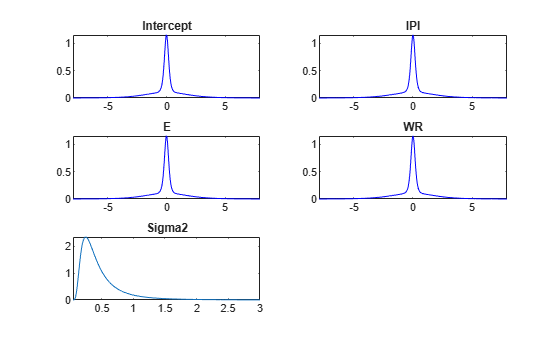The prior distribution of each coefficient is a mixture of two Gaussians: both components have a mean of zero, but component 1 has a large variance relative to component 2. Therefore, their distributions are centered at zero and have the spike-and-slab appearance.

Consider the linear regression model in Create Prior Model for SSVS.

Create a prior model for performing SSVS. Assume that $\beta$ and ${\sigma }^{2}\text{\hspace{0.17em}}$ are dependent (a conjugate mixture model). Specify the number of predictors p and the names of the regression coefficients.

p = 3; PriorMdl = mixconjugateblm(p,'VarNames',["IPI" "E" "WR"]);

Display the prior regime probabilities and Gaussian mixture variance factors of the prior $\beta$.

priorProbabilities = table(PriorMdl.Probability,'RowNames',PriorMdl.VarNames,... 'VariableNames',"Probability")
priorProbabilities=4×1 table Probability ___________ Intercept 0.5 IPI 0.5 E 0.5 WR 0.5 
priorV = array2table(PriorMdl.V,'RowNames',PriorMdl.VarNames,... 'VariableNames',["gammaIs1" "gammaIs0"])
priorV=4×2 table gammaIs1 gammaIs0 ________ ________ Intercept 10 0.1 IPI 10 0.1 E 10 0.1 WR 10 0.1 

PriorMdl stores prior regime probabilities in the Probability property and the regime variance factors in the V property. The default prior probability of variable inclusion is 0.5. The default variance factors for each coefficient are 10 for the variable-inclusion regime and 0.01 for the variable-exclusion regime.

Load the Nelson-Plosser data set. Create variables for the response and predictor series.

load Data_NelsonPlosser X = DataTable{:,PriorMdl.VarNames(2:end)}; y = DataTable{:,'GNPR'};

Implement SSVS by estimating the marginal posterior distributions of $\beta$ and ${\sigma }^{2}$. Because SSVS uses Markov chain Monte Carlo (MCMC) for estimation, set a random number seed to reproduce the results.

rng(1); PosteriorMdl = estimate(PriorMdl,X,y);
Method: MCMC sampling with 10000 draws Number of observations: 62 Number of predictors: 4 | Mean Std CI95 Positive Distribution Regime ---------------------------------------------------------------------------------- Intercept | -18.8333 10.1851 [-36.965, 0.716] 0.037 Empirical 0.8806 IPI | 4.4554 0.1543 [ 4.165, 4.764] 1.000 Empirical 0.4545 E | 0.0010 0.0004 [ 0.000, 0.002] 0.997 Empirical 0.0925 WR | 2.4686 0.3615 [ 1.766, 3.197] 1.000 Empirical 0.1734 Sigma2 | 47.7557 8.6551 [33.858, 66.875] 1.000 Empirical NaN 

PosteriorMdl is an empiricalblm model object that stores draws from the posterior distributions of $\beta$ and ${\sigma }^{2}$ given the data. estimate displays a summary of the marginal posterior distributions at the command line. Rows of the summary correspond to regression coefficients and the disturbance variance, and columns correspond to characteristics of the posterior distribution. The characteristics include:

• CI95, which contains the 95% Bayesian equitailed credible intervals for the parameters. For example, the posterior probability that the regression coefficient of E (standardized) is in [0.000, 0.002] is 0.95.

• Regime, which contains the marginal posterior probability of variable inclusion ($\gamma =1$ for a variable). For example, the posterior probability that E should be included in the model is 0.0925.

Assuming, that variables with Regime < 0.1 should be removed from the model, the results suggest that you can exclude the unemployment rate from the model.

By default, estimate draws and discards a burn-in sample of size 5000. However, a good practice is to inspect a trace plot of the draws for adequate mixing and lack of transience. Plot a trace plot of the draws for each parameter. You can access the draws that compose the distribution (the properties BetaDraws and Sigma2Draws) using dot notation.

figure; for j = 1:(p + 1) subplot(2,2,j); plot(PosteriorMdl.BetaDraws(j,:)); title(sprintf('%s',PosteriorMdl.VarNames{j})); end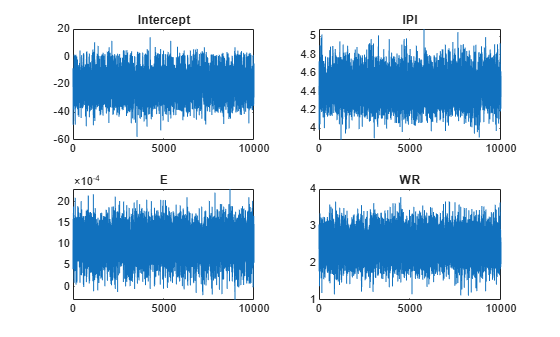figure; plot(PosteriorMdl.Sigma2Draws); title('Sigma2');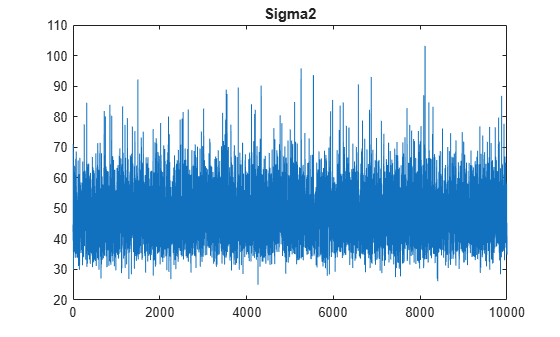The trace plots indicate that the draws seem to mix well. The plots show no detectable transience or serial correlation, and the draws do not jump between states.

Consider the linear regression model in Create Prior Model for SSVS.

Load the Nelson-Plosser data set. Create variables for the response and predictor series. Add example-specific files to the MATLAB® path.

load Data_NelsonPlosser VarNames = ["IPI" "E" "WR"]; X = DataTable{:,VarNames}; y = DataTable{:,"GNPR"}; path = fullfile(matlabroot,'examples','econ','main'); addpath(path); 

Assume the following:

• The intercept is in the model with probability 0.9.

• IPI and E are in the model with probability 0.75.

• If E is included in the model, then the probability that WR is included in the model is 0.9.

• If E is excluded from the model, then the probability that WR is included is 0.25.

Declare a function named priorssvsexample.m that:

• Accepts a logical vector indicating whether the intercept and variables are in the model (true for model inclusion). Element 1 corresponds to the intercept, and the rest of the elements correspond to the variables in the data.

• Returns a numeric scalar representing the log of the described prior regime probability distribution.

function logprior = priorssvsexample(varinc) %PRIORSSVSEXAMPLE Log prior regime probability distribution for SSVS % PRIORSSVSEXAMPLE is an example of a custom log prior regime probability % distribution for SSVS with dependent random variables. varinc is % a 4-by-1 logical vector indicating whether 4 coefficients are in a model % and logPrior is a numeric scalar representing the log of the prior % distribution of the regime probabilities. % % Coefficients enter a model according to these rules: % * varinc(1) is included with probability 0.9. % * varinc(2) and varinc(3) are in the model with probability 0.75. % * If varinc(3) is included in the model, then the probability that % varinc(4) is included in the model is 0.9. % * If varinc(3) is excluded from the model, then the probability % that varinc(4) is included is 0.25. logprior = log(0.9) + 2*log(0.75) + log(varinc(3)*0.9 + (1-varinc(3))*0.25); end 

priorssvsexample.m is an example-specific file included with Econometrics Toolbox™. To access it, enter edit priorssvsexample.m at the command line.

Create a prior model for performing SSVS. Assume that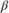and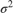are (a conjugate mixture model). Specify the number of predictors p the names of the regression coefficients, and custom, prior probability distribution of the variable-inclusion regimes.

p = 3; PriorMdl = mixconjugateblm(p,'VarNames',["IPI" "E" "WR"],... 'Probability',@priorssvsexample); 

Implement SSVS by estimating the marginal posterior distributions ofand. Because SSVS uses MCMC for estimation, set a random number seed to reproduce the results.

rng(1); PosteriorMdl = estimate(PriorMdl,X,y); 
Method: MCMC sampling with 10000 draws Number of observations: 62 Number of predictors: 4 | Mean Std CI95 Positive Distribution Regime ---------------------------------------------------------------------------------- Intercept | -18.7971 10.1644 [-37.002, 0.765] 0.039 Empirical 0.8797 IPI | 4.4559 0.1530 [ 4.166, 4.760] 1.000 Empirical 0.4623 E | 0.0010 0.0004 [ 0.000, 0.002] 0.997 Empirical 0.2665 WR | 2.4684 0.3618 [ 1.759, 3.196] 1.000 Empirical 0.1727 Sigma2 | 47.7391 8.6741 [33.823, 67.024] 1.000 Empirical NaN 

Assuming, that variables with Regime < 0.1 should be removed from the model, the results suggest that you can include all variables in the model.

Because this example requires path to access example-specific files, clean up by removing path from the MATLAB® path.

rmpath(path); 

Consider the regression model in Create Prior Model for SSVS.

Perform SSVS:

1. Create a Bayesian regression model for SSVS with a conjugate prior for the data likelihood. Use the default settings.

2. Hold out the last 10 periods of data from estimation.

3. Estimate the marginal posterior distributions.

p = 3; PriorMdl = bayeslm(p,'ModelType','mixconjugate','VarNames',["IPI" "E" "WR"]); load Data_NelsonPlosser fhs = 10; % Forecast horizon size X = DataTable{1:(end - fhs),PriorMdl.VarNames(2:end)}; y = DataTable{1:(end - fhs),'GNPR'}; XF = DataTable{(end - fhs + 1):end,PriorMdl.VarNames(2:end)}; % Future predictor data yFT = DataTable{(end - fhs + 1):end,'GNPR'}; % True future responses rng(1); % For reproducibility PosteriorMdl = estimate(PriorMdl,X,y,'Display',false);

Forecast responses using the posterior predictive distribution and the future predictor data XF. Plot the true values of the response and the forecasted values.

yF = forecast(PosteriorMdl,XF); figure; plot(dates,DataTable.GNPR); hold on plot(dates((end - fhs + 1):end),yF) h = gca; hp = patch([dates(end - fhs + 1) dates(end) dates(end) dates(end - fhs + 1)],... h.YLim([1,1,2,2]),[0.8 0.8 0.8]); uistack(hp,'bottom'); legend('Forecast Horizon','True GNPR','Forecasted GNPR','Location','NW') title('Real Gross National Product: 1909 - 1970'); ylabel('rGNP'); xlabel('Year'); hold off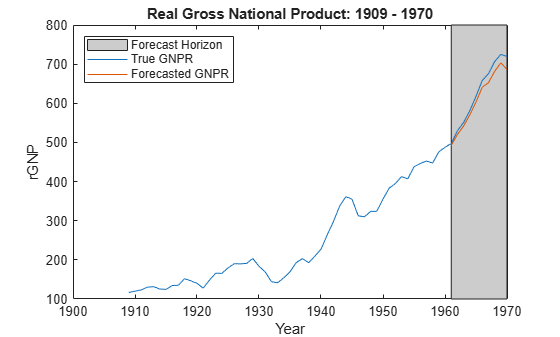yF is a 10-by-1 vector of future values of real GNP corresponding to the future predictor data.

Estimate the forecast root mean squared error (RMSE).

frmse = sqrt(mean((yF - yFT).^2))
frmse = 18.8470 

The forecast RMSE is a relative measure of forecast accuracy. Specifically, you estimate several models using different assumptions. The model with the lowest forecast RMSE is the best-performing model of the ones being compared.

When you perform Bayesian regression with SSVS, a best practice is to tune the hyperparameters. One way to do so is to estimate the forecast RMSE over a grid of hyperparameter values, and choose the value that minimizes the forecast RMSE.

expand all

## Algorithms

A closed-form posterior exists for conjugate mixture priors in the SSVS framework with K coefficients. However, because the prior β|σ2,γ, marginalized by γ, is a 2K-component Gaussian mixture, MATLAB® uses MCMC instead to sample from the posterior for numerical stability.

## Alternative Functionality

The bayeslm function can create any supported prior model object for Bayesian linear regression.

 George, E. I., and R. E. McCulloch. "Variable Selection Via Gibbs Sampling." Journal of the American Statistical Association. Vol. 88, No. 423, 1993, pp. 881–889.

 Koop, G., D. J. Poirier, and J. L. Tobias. Bayesian Econometric Methods. New York, NY: Cambridge University Press, 2007.